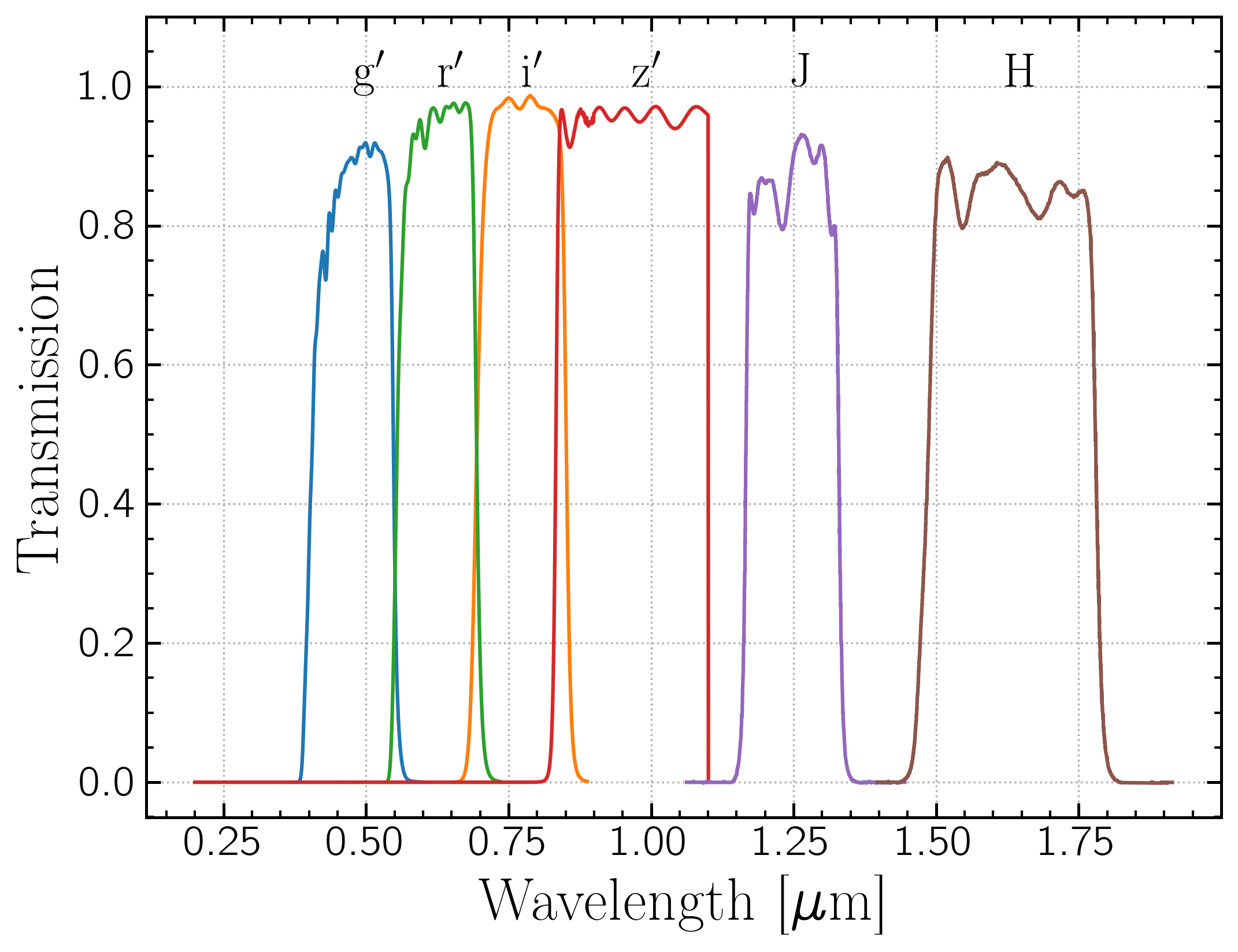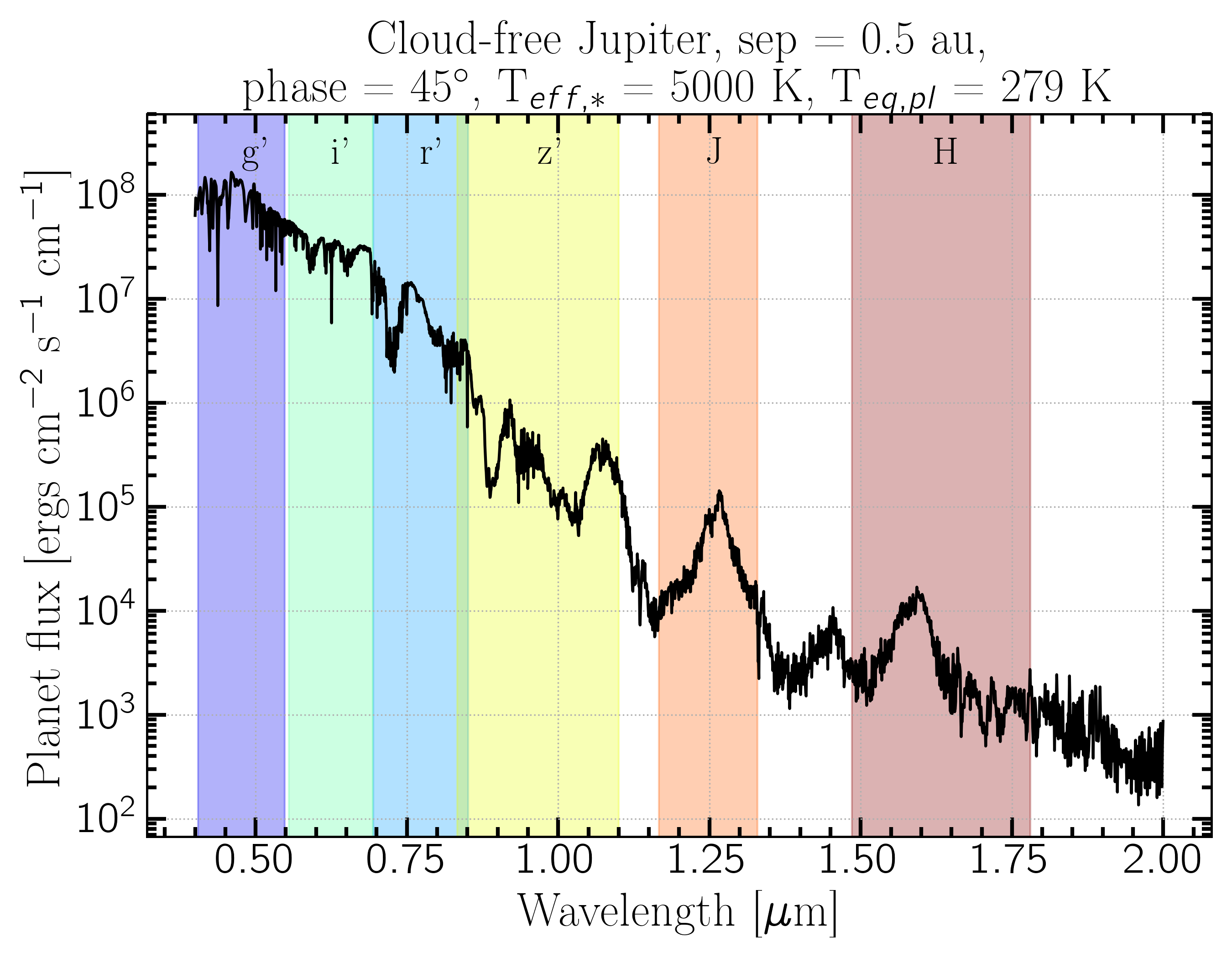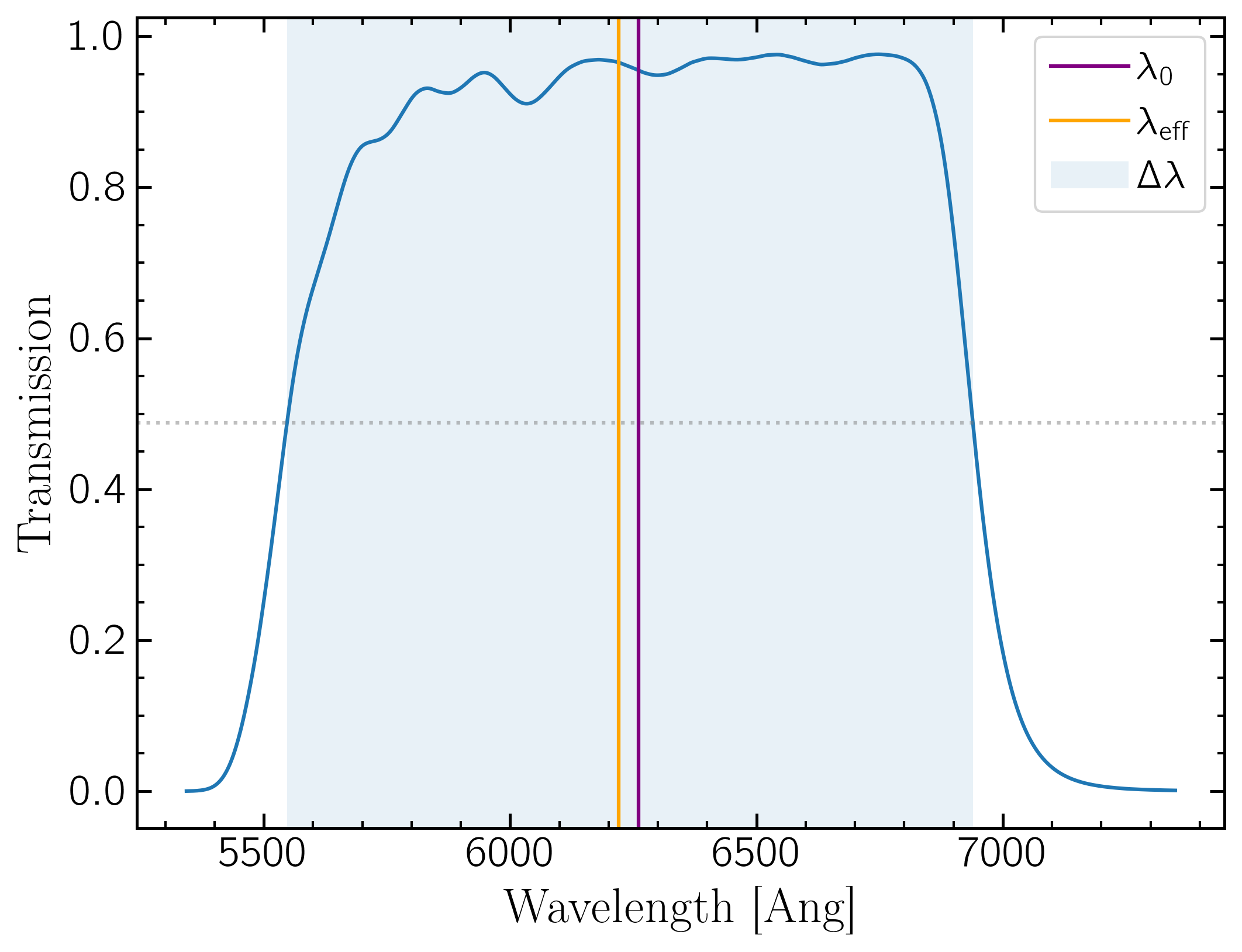Every time I go to work with photometry I get very confused about wavelengths, spectra filter curves, and how to get useful quantities like flux and magnitudes. I am writing this explainer to future me.

Ok so here are some filter transmission curves:And an example reflected light model spectrum:I will use this spectrum for all discussion and calculations throughout. It was produced using PICASO, a 1d self-consistent radiative transfer code for modeling exoplanet atmospheres. So the flux shown is from the surface of the star. So the first thing to do is correct for the flux observed from Earth. $$F_{\lambda} (\lambda) = I (\lambda) \; \Omega$$ $$\Omega = \frac{\pi R_p^2}{D^2}$$ where $I$ is the intensity at the planet surface, D is the distance to the planet, and R$_p$ is the radius of the planet. Of course distance and radius must be in the same unit, which you can do easily with astropy
                                
import astropy.units as u
D = 10*u.pc
Rp = 1*u.Rjup
Omega = np.pi * ((Rp/D).decompose())**2


Don't forget to pay attention to the flux units. I am using $f_\lambda$ throughout. If you need to convert from $f_\nu$, such as Janskys, it's: $$f_\nu = \frac{\lambda^2}{c} f_\lambda$$
or $$\frac{f_\nu}{\rm{Jy}} = 3.34\times10^4\; \left(\frac{\lambda}{\rm{Ang}}\right)^2\; \frac{f_\lambda}{\rm{ergs}\; cm^{-2}\; s^{-1}\; Ang^{-1}}$$

## Combining filters and spectra

Now to convolve the spectrum with the filter curve. There are two conventions (to my knowledge) for reporting flux in a filter: the average flux in the filter and the flux at the central wavelength $\lambda_0$

### Flux at $\lambda_0$:

$$\lambda_0 = \frac{\int_{0}^{\infty} \lambda \; R(\lambda)\,d\lambda}{\int_{0}^{\infty} R(\lambda)\,d\lambda}$$
where $R(\lambda)$ is the filter transmission curve. It's basically the weighted average wavelength of the filter curve. It's often called central wavelength. There is also the pivot" or effective wavelength, which is $$\lambda_{\rm{eff}}^2 = \frac{\int_{0}^{\infty} R(\lambda)\,d\lambda}{\int_{0}^{\infty} \frac{1}{\lambda^2} R(\lambda)\,d\lambda}$$ This is the value of $\lambda$ that makes the conversion in the flux conversion equation exact for that filter(reference). Sometimes $\lambda_0$ is also called effective width. It can be confusing which definition people are using when they use eff width without defining how it is computed. But they are really close in value so it often makes little difference. I will use $\lambda_0$ or central wavelength to be precise.

Now $$F_\lambda(\lambda_0)\; \Delta \lambda = \int_{0}^{\infty} F_\lambda(\lambda)\; R(\lambda)\,d\lambda$$ where $\Delta \lambda$ is the effective width, or the full width at half maximum (FWHM).
An illustration of these definitions:Because filters have all different shapes, the average flux in the filter can't be written as the flux at the central wavelength. So the convention is typically to report the flux at the central wavelength as the flux in the filter. This will require interpolating the flux array to get the flux at the central wavelength. One way to do it is:
                                
from scipy.interpolate import interp1d
# create the interpolation function:
interpfunc = interp1d(spectrum_wavelength,spectrum_flux, fill_value="extrapolate")
# Interpolate the filter's central wavelength in the spectrum's flux array:
F_lambda_0 = interpfunc(lambda_0)


Pysynphot also has an easy interface for sampling a "source spectrum" object at a specific wavelength.

This is a good way to go for computing colors in different filters since it accounts for the difference in filter widths for the two filters without having to compute them.

### Average Filter Flux:

Another way to do it is compute the weighted average of the flux multiplied by the filter curve. $$\overline{F_\lambda(\lambda)} = \frac{\sum F_\lambda(\lambda) \times R(\lambda)}{ \sum R(\lambda)}$$ In code this can be done:
                                
from scipy.interpolate import interp1d
import numpy as np
### We need to interpolate the spectrum's flux array onto the filter's wavelength array so they can be multiplied:
# First cut off areas of spectrum outside the filter curve to avoid interpolation errors:
ind = np.where((spectrum_wavelength > np.min(filter_wavelength)) & spectrum_wavelength < np.max(filter_wavelength)))
# Make interpolation function:
interpfunc = interp1d(spectrum_wavelength[ind],spectrum_flux[ind], fill_value="extrapolate")
# interpolate the spectrum's flux on the filter's wavelength array:
flux_on_filter_wavelength_grid = interpfunc(filter_wavelength)
# Multiply flux by filter transmission:
filter_times_flux = flux_on_filter_wavelength_grid * filter_transmission
# Compute weighted average:
filter_weighted_average = np.sum(filter_times_flux) / np.sum(filter_transmission)


Again Pysynphot has an easy interface for combining filter curves and spectra as well.

But this is not the same as the flux at the central wavelength, so it is better plotted as a bar spanning the filter's $\Delta \lambda$ rather than as a point at a specific wavelength.
This is a good metric to use if you're trying to account for the total flux or energy received in a filter.

### Comparing them:

The two methods are close but aren't exactly the same. This figure shows the two methods applied to the spectrum shown above. Because of spectrum features the two conventions typically don't agree. In the i$^{\prime}$, $\lambda_0$ happens to fall on an absorption feature and so is lower than the average, while in J band, absorption features on either side of $\lambda_0$ make F($\lambda_0$) much higher than the average. Coincidentally they are almost the same in H band for this model. So neither are correct" (whatever that means here), but both are used. It's important to define which one is being used and plot them appropriately.